#### You may also like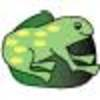### The Lily Pond

Freddie Frog visits as many of the leaves as he can on the way to see Sammy Snail but only visits each lily leaf once. Which is the best way for him to go?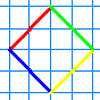### A Cartesian Puzzle

Find the missing coordinates which will form these eight quadrilaterals. These coordinates themselves will then form a shape with rotational and line symmetry.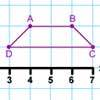### Transformation Tease

What are the coordinates of this shape after it has been transformed in the ways described? Compare these with the original coordinates. What do you notice about the numbers?

# Fred the Class Robot

##### Age 7 to 11Challenge Level

Billy's school has a paved area at the front: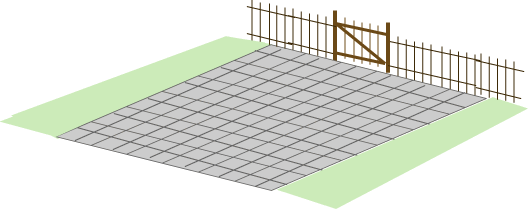Billy's class had a robot called Fred who could draw with chalk held underneath him.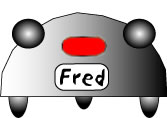Billy's teacher drew lines and numbers on the paved area in front of the school so that they could tell Fred where to go.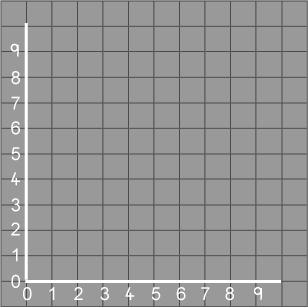Billy's teacher put a little cross on the point $(1, 2)$ and the children put Fred on the cross. Fred moved to $(4, 7)$, then to $(4, 2)$ and then back to $(1, 2 )$. Fred's chalk drew a line to show where he had been.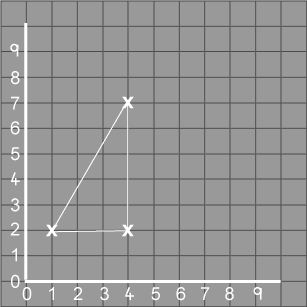"What shape has Fred drawn?" asked Billy's teacher.
"A triangle!" shouted all the class.
All the children wanted to make Fred draw shapes.
Tom had the first turn. He got Fred to go from $(6, 1)$ to $(6, 6)$, and then to $(1, 6)$ and $(1, 1)$ and back to $(6, 1)$.
Vicky made Fred go from $(2, 1)$ to $(7, 2)$, and then to $(9, 4)$ and $(4, 6)$ and then $(1, 5)$ and back to $(2, 1)$.
Billy thought very hard when it was his turn. He made Fred go from $(7, 4)$ to $(4, 7)$, and then to $(1, 4)$ and $(4, 1)$ and back to $(7, 4)$.
If Billy had made Fred start at the same place, $(7, 4)$, how else could a shape like this be drawn on the $9$ by $9$ axes? (It doesn't have to be the same size as Billy's first shape.)